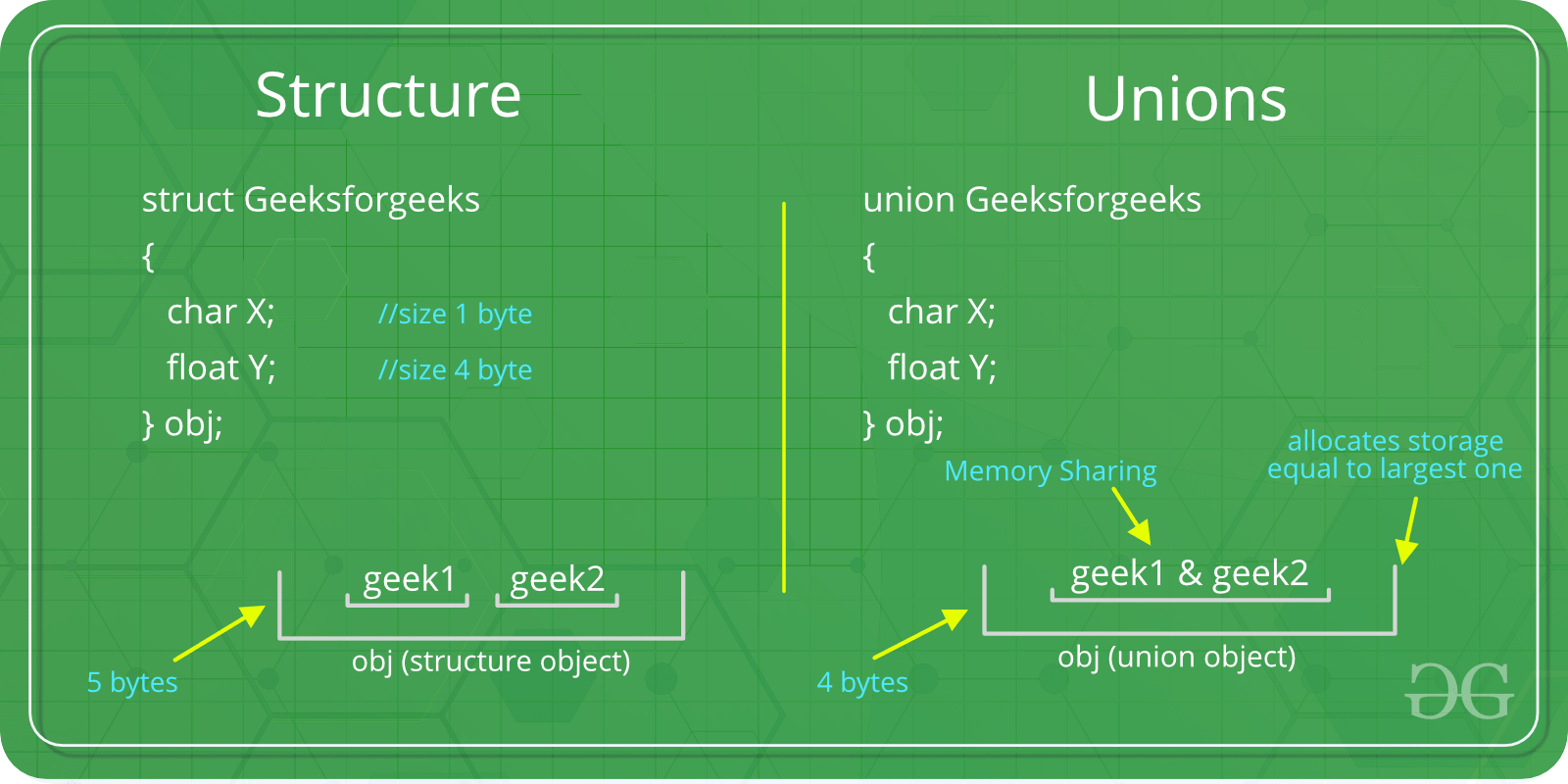# Union in C

• Difficulty Level : Easy
• Last Updated : 08 Feb, 2019

Like Structures, union is a user defined data type. In union, all members share the same memory location.For example in the following C program, both x and y share the same location. If we change x, we can see the changes being reflected in y.

 `#include `` ` `// Declaration of union is same as structures``union` `test {``    ``int` `x, y;``};`` ` `int` `main()``{``    ``// A union variable t``    ``union` `test t;`` ` `    ``t.x = 2; ``// t.y also gets value 2``    ``printf``(``"After making x = 2:\n x = %d, y = %d\n\n"``,``           ``t.x, t.y);`` ` `    ``t.y = 10; ``// t.x is also updated to 10``    ``printf``(``"After making y = 10:\n x = %d, y = %d\n\n"``,``           ``t.x, t.y);``    ``return` `0;``}`

Output:

```After making x = 2:
x = 2, y = 2

After making y = 10:
x = 10, y = 10
```

How is the size of union decided by compiler?
Size of a union is taken according the size of largest member in union.

 `#include `` ` `union` `test1 {``    ``int` `x;``    ``int` `y;``} Test1;`` ` `union` `test2 {``    ``int` `x;``    ``char` `y;``} Test2;`` ` `union` `test3 {``    ``int` `arr;``    ``char` `y;``} Test3;`` ` `int` `main()``{``    ``printf``(``"sizeof(test1) = %lu, sizeof(test2) = %lu, "``           ``"sizeof(test3) = %lu"``,``           ``sizeof``(Test1),``           ``sizeof``(Test2), ``sizeof``(Test3));``    ``return` `0;``}`

Output:

```sizeof(test1) = 4, sizeof(test2) = 4, sizeof(test3) = 40
```

Pointers to unions?
Like structures, we can have pointers to unions and can access members using the arrow operator (->). The following example demonstrates the same.

 `#include `` ` `union` `test {``    ``int` `x;``    ``char` `y;``};`` ` `int` `main()``{``    ``union` `test p1;``    ``p1.x = 65;`` ` `    ``// p2 is a pointer to union p1``    ``union` `test* p2 = &p1;`` ` `    ``// Accessing union members using pointer``    ``printf``(``"%d %c"``, p2->x, p2->y);``    ``return` `0;``}`

Output:

```65 A
```

What are applications of union?
Unions can be useful in many situations where we want to use the same memory for two or more members. For example, suppose we want to implement a binary tree data structure where each leaf node has a double data value, while each internal node has pointers to two children, but no data. If we declare this as:

 `struct` `NODE {``    ``struct` `NODE* left;``    ``struct` `NODE* right;``    ``double` `data;``};`

then every node requires 16 bytes, with half the bytes wasted for each type of node. On the other hand, if we declare a node as following, then we can save space.

 `struct` `NODE {``    ``bool` `is_leaf;``    ``union` `{``        ``struct``        ``{``            ``struct` `NODE* left;``            ``struct` `NODE* right;``        ``} internal;``        ``double` `data;``    ``} info;``};`

The above example is taken from Computer Systems : A Programmer’s Perspective (English) 2nd Edition book.